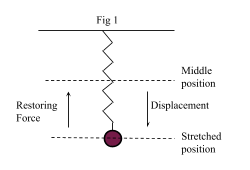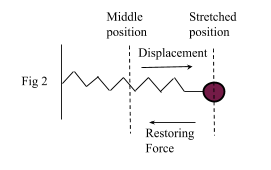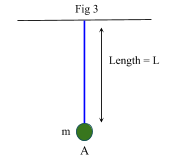# Simple Harmonic Motion

Contents

## Summary

• Simple harmonic motion is any motion where the acceleration of restoring force is directly proportional to its displacement.
• Simple harmonic motion is defined by the formula acceleration$=\quad -{ \omega }^{ 2 }x$.
• The period of oscillation in simple harmonic motion is given by the formula$T\quad =\quad \frac { 2\pi }{ \omega }$.
• Simple pendulum is also a simple harmonic motion as we showed that by resolving 2 rectangular components of weight of the bob, it is proved that acceleration of the restoring force is proportional to its displacement.

### Simple Harmonic Motion

Simple harmonic motion is any motion where a restoring force is applied that is proportional to the displacement and in the opposite direction of that displacement.

A very common example of simple harmonic motion is a mass or particle attached to a spring, as more the particle is stretched or pulled, the more it experiences a force that pulls it back to the rest position which means it accelerates backwards.

This scenario could either be vertical in which case gravity is involved as shown in Fig 1 or horizontal on a flat surface as shown in Fig 2.For an oscillation particle like the one discussed above, let displacement = x, and its acceleration defined by the equation:$-{ \omega }^{ 2 }x$

Where 2 is a positive constant. This equation proves that acceleration of the restoring force is directly proportional to the displacement.

We have seen the equation of simple harmonic motion in terms of acceleration and displacement.

Next we will derive the equation of simple harmonic motion in terms of velocity v and displacement x.

We know:$\frac { { d }^{ 2 }x }{ d{ t }^{ 2 } } \quad =\quad -{ \omega }^{ 2 }x\quad \quad \Rightarrow \quad equation1$$Acceleration\quad =\quad \frac { { d }^{ 2 }x }{ d{ t }^{ 2 } } \quad =\quad \frac { dv }{ dt }$$Velocity\quad =\quad v\quad =\quad \frac { dx }{ dt }$

Therefore:$\frac { dv }{ dt } \quad =\quad \frac { dv }{ dx } \quad \times \quad \frac { dx }{ dt }$$\frac { dv }{ dt } \quad =\quad v\quad \frac { dv }{ dx }$

Substitute this expression into equation 1:$\frac { { d }^{ 2 }x }{ d{ t }^{ 2 } } \quad =\quad \frac { dv }{ dt } \quad =\quad -{ \omega }^{ 2 }x$$v\quad \frac { dv }{ dx } \quad =\quad -{ \omega }^{ 2 }x$

Separate the variables so we are able to integrate the expression$\int { v\quad dv\quad =\quad \int { -{ \omega }^{ 2 }x\quad dx } }$$\frac { 1 }{ 2 } { v }^{ 2 }\quad =\quad -\frac { 1 }{ 2 } { { \omega }^{ 2 }x }^{ 2 }\quad +\quad C$

a is the oscillation amplitude, hence:${ v }^{ 2 }\quad =\quad { { \omega }^{ 2 } }({ a }^{ 2 }\quad -\quad { x }^{ 2 })$

When x = a, velocity becomes 0$v\quad =\quad \pm \quad \omega \sqrt { ({ a }^{ 2 }\quad -\quad { x }^{ 2 }) }$

Now further integrating this expression will give us an equation for the displacement with respect to time which is:$x\quad =\quad a\quad cos(\omega t)$

The simple harmonic oscillator completes one oscillation whenever it covers twice the end-to-end distance for example if the amplitude of oscillation is a. Then twice the end- to-end distance would mean 4a.

This is one complete revolution and thus, the period of oscillation in simple harmonic motion is given by:$T\quad =\quad \frac { 2\pi }{ \omega }$

#### Example #1

Q. A road drill vibrates up and down with SHM at a frequency of 20 Hz. What’s the maximum acceleration of the pick head if the amplitude of the oscillation is 5 cm?

Solution:

We know: f = 20 Hz and a = 5 cm

From the given frequency we can find the value of$\omega$  (omega):$f\quad =\quad \frac { 1 }{ T } \quad =\quad \frac { 1 }{ 20 }$

We know$T\quad =\quad \frac { 2\pi }{ \omega }$,  therefore:$20\quad =\quad \frac { 2\pi }{ \omega }$$\omega \quad =\quad \frac { \pi }{ 10 }$

Now that we have found the value of , we can use the formula to find maximum acceleration:$Acceleration\quad =\quad -{ \omega }^{ 2 }x$$=\quad -{ (\frac { \pi }{ 10 } ) }^{ 2 }\frac { 5 }{ 100 }$$=\quad790\quad m{ s }^{ -2 }$        Ans

### Simple Pendulum

A simple pendulum also exhibits Simple harmonic motion. It consists of a small bob of mass m suspended from a light string of length L fixed at its upper end as shown in Fig 3.In the equilibrium position (position A), the bob is at rest and the net force on the bob is zero.

Now if we bring the bob to a new position B as shown in Fig 4, where the angle formed is$x\quad =\quad \theta$,  then the net force is no longer zero. Due to the weight of the bob we will have a vertical and a horizontal component of force acting on the bob.The weight of the bob will be equal to mg where g is the gravitational acceleration.

The two components of weight are:

• mg cos (θ) = component of weight along the string
• mg sin (θ) = component of the weight perpendicular to the string.

To resolve the vertical component first, we see that tension in the string T is equal to the vertical component of the weight as the bob is stationary at this point.$T\quad =\quad mg\quad cos\theta \quad \quad \quad \quad \Rightarrow \quad \quad \quad equation\quad1$

As these two forces balance each other, hence the vertical component has no contribution in the motion of the bob.

Next we move onto the horizontal component. As the horizontal component of the weight mg sin (θ) causes a restoring force which pulls the bob back to its initial position, therefore, this can be resolved by forming an equation;$F\quad =\quad -mg\quad sin\theta \quad \quad \quad \quad \Rightarrow \quad \quad \quad equation\quad 2$

Since:

F = m a

We can write equation 2 as:$ma\quad =\quad -mg\quad sin\theta$$a\quad =\quad -g\theta \quad (when\quad \theta \quad is\quad small\quad sin\theta \quad =\quad \theta )\quad \quad \quad \quad \Rightarrow \quad \quad \quad equation\quad 3$

As the extended pendulum makes an arc, we can use the formula of arc length:

s  =  r$\theta$ , in this case r = L and arc length s = x$\theta \quad =\quad \frac { x }{ L }$

Substitute in equation 3$a\quad =\quad -g\frac { x }{ L }$

Therefore, this proves that simple pendulum is also a simple harmonic motion as acceleration a is directly proportional to displacement x.